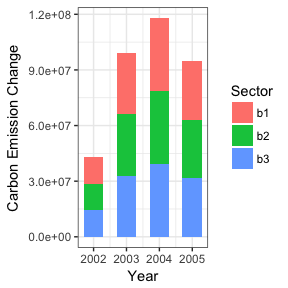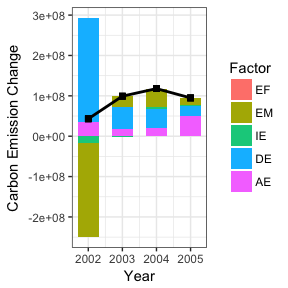# Energy Decomposition Analysis Using EDA R Package

#### 2018-07-02

Energy decomposition analysis is used for measuring contributions of components and factors on energy or carbon emission changes (Ang 2005, Marlay 1984, Liu et al. 2003).

EDA package consists of five energy consumption analysis methods: Log Mean Devisia Index (LMDI) method (Ang 2005), Laspeyres method, Paasche method (Paasche 1874), Marshall-Edgeworth method (Marshall 1887, Edgeworth 1925) and Walsh method (Walsh 1921). The formulas of five methods are listed in Table 1.

Table 1.

Method use in EDA function Formula
LMDI method = "LMDI" $${\Delta} C_{x_{1}} = \sum_{i} L\left(C_{i}^{T}, C_{i}^{0}\right) \ln\frac{x_{i}^{T}}{x_{i}^{0}} = \sum_{i}\frac{C_{i}^{T}-C_{i}^{0}}{\ln C_{i}^{T} - \ln C_{i}^{0}} \ln\frac{x_{i}^{T}}{x_{i}^{0}}$$
Laspeyres method = "Laspeyres" $${\Delta} C_{x_{1}} = \sum_{i} \left(x_{1i}^{T} - x_{1i}^{0}\right) x_{2i}^{0} ... x_{ni}^{0}$$
Paasche method = "Paasche" $${\Delta} C_{x_{1}} = \sum_{i} \left(x_{1i}^{T} - x_{1i}^{0}\right) x_{2i}^{T} ... x_{ni}^{T}$$
Marshall-Edgeworth method = "Marshall-Edgeworth" $${\Delta} C_{x_{1}} = \sum_{i} \left(x_{1i}^{T} - x_{1i}^{0}\right) \frac{x_{2i}^{T} + x_{2i}^{0}}{2} ... \frac{x_{ni}^{T} + x_{ni}^{0}}{2}$$
Walsh method = "Walsh" $${\Delta} C_{x_{1}} = \sum_{i} \left(x_{1i}^{T} - x_{1i}^{0}\right) \sqrt{x_{2i}^{T} x_{2i}^{0}} ... \sqrt{x_{ni}^{T} x_{ni}^{0}}$$

where

$${\Delta} C_{x_{1}}$$ is carbon emission change due to the change of factor $${x_{1}}$$;

$$i$$ is the subscript of a certain type of fuel or industrial sector;

$$L(a,b)=\frac{a-b}{\ln{a} - \ln{b}}$$ is a function defined by Ang (2005);

$$C_{i}^{0}$$ and $$C_{i}^{T}$$ are carbon emissions at a former time $$T$$ (e.g. the first year) and a later time $$0$$ (e.g. the second year), respectively;

$$x_{1i}^{0}$$ and $$x_{1i}^{T}$$ are factor values of $$x_{1i}$$ at a former time $$T$$ (e.g. the first year) and a later time $$0$$ (e.g. the second year), respectively.

EDA package includes a CarbonEmission dataset, which consists of:

• carbon: A data frame of carbon emissions from four types of fuels and three building sectors;

• energy: A data frame of energy consumption from four types of fuels and three building sectors;

• buildingarea: A data frame of annual building areas.

• factordata: A list of data frames of factors of carbon emission changes.

Further information can be found on the manual of EDA package.

## Converting variables to carbon emission factors

Generally, carbon emission factors are computed from a series of variables in terms of definitions of factors and research objectives. This section introduces the process of converting variables to carbon emission factors using the CarbonEmission dataset provided in the EDA package. If your factors data are available, please go to the next section. The result is the factors dataset factordata. If you want to directly try the EDA and LMDI functions, you can load the dataset factordata.

library("EDA")
data("carbon")
data("energy")
data("buildingarea")
#>   year buildingsector     fuel1    fuel2    fuel3    fuel4
#> 1 2001             b1 112352430 16183936 32441629 922497.0
#> 2 2002             b1 122822812 18221536 34264130 943390.2
#> 3 2003             b1 148904464 21525776 37958976 922106.2
# calculate factors data
## Factor 1: Emission-factor effect (EF)
cdata <- carbon[,-c(1,2)]
edata <- energy[,-c(1,2)]
Sector <- c("b1", "b2", "b3")
xdata1 <- cdata/edata
## Factor 2: Energy-mix effect (EM)
Esum <- aggregate(edata, by=list(energy[,1]), FUN=sum, na.rm = TRUE)[,-1]
Esum <- Esum[rep(seq_len(nrow(Esum)), length(Sector)), ]
xdata2 <- edata/Esum
## Factor 3: Intensity effect (IE)
e_yearsum <- rowSums(Esum)
xdata3 <- Esum/e_yearsum
## Factor 4: Energy consumption density effect (DE)
area <- rep(buildingarea[,2], length(Sector))
xdata4 <- e_yearsum/area
xdata4 <- as.data.frame(xdata4)
## Factor 5: Area effect (AE)
xdata5 <- as.data.frame(area)
## list of factors
factordata <- list(xdata1, xdata2, xdata3, xdata4, xdata5)
names(factordata) <- c("EF","EM","IE","DE","AE")

## Log Mean Devisia Index (LMDI) method: LMDI

data(carbon)
data(factordata)
## set parameters
cdata <- carbon[,-c(1,2)]
C0 <- cdata[1,]
CT <- cdata[2,]
X0 <- factordata[][1,]
XT <- factordata[][2,]
## run LMDI model
ed1 <- LMDI(C0, CT, X0, XT)
ed1
#> Total change:  -77294208
#> Change by fuel types:
#>         fuel1         fuel2         fuel3         fuel4
#> -77662850.340    373443.052     -1112.859     -3688.288

Further information can be found on the manual of EDA package.

## Energy consumption analysis: EDA

data(carbon)
data(factordata)
## set parameters
cdata <- carbon[,-c(1,2)]
Year <- 2001:2005
Sector <- c("b1", "b2", "b3")
Fuel <- colnames(cdata)
Factor <- names(factordata)
## run EDA model
eda1 <- EDA(cdata, factordata, Year = Year, Factor = Factor,
Fuel = Fuel, Sector = Sector, method = "LMDI")
eda1
#> Annual changes:
#>   Year    Change
#> 1 2002  43054129
#> 2 2003  99178362
#> 3 2004 117880293
#> 4 2005  94842144
#> Total change:  354954928
plot(eda1)## Reference

Song Y (2018). EDA: Energy Decomposition Analysis. R package, https://CRAN.R-project.org/package=EDA.

Ang, B. W. (2005). The LMDI approach to decomposition analysis: a practical guide. Energy policy, 33(7), 867-871. doi: 10.1016/j.enpol.2003.10.010.

Liu, F. L., & Ang, B. W. (2003). Eight methods for decomposing the aggregate energy-intensity of industry. Applied Energy, 76(1-3), 15-23.

Marlay, R. C. (1984). Trends in industrial use of energy. Science, 226, 1277-1284.

Paasche, H. (1874). Uber die Preisentwicklung der letzten Jahre. Jahrbiicher fur Nationalokonomie und Statistik, 23, 168.

Marshall, A. (1887). Remedies for fluctuations of general prices.

Edgeworth, F. Y. (1925). Papers relating to political economy.

Walsh, C. M. (1921). The Problem of Estimation, a Seventeenth-century Controversy and Its Bearing on Modern Statistical Questions, Especially Index-numbers, by Correa Moylan Walsh.

## Author and Contact

Yongze Song

Curtin University, Australia